• 学习中心极限定理，学习正态分布，学习最大似然估计 最优化理论及方法 高等数学 统计学 概率论 参数估计 正态分布中心极限定理(中心极限定理正态分布的一个前置知识) 如果误差可以看作许多微小量...


学习中心极限定理，学习正态分布，学习最大似然估计

最优化理论及方法

高等数学

统计学

概率论

参数估计

正态分布与中心极限定理(中心极限定理是正态分布的一个前置知识)

如果误差可以看作许多微小量的叠加，则根据中心极限定理(用样本的平均值估计总体的期望)，随机误差理所当然服从正态分布；

假设一随机变量X服从一个期望和方差分别为
$\mu{和}\sigma^2$
的正态分布，概率密度函数为
$f(x)=\frac{1}{\sqrt{2 \pi} \sigma} \exp \left(-\frac{(x-\mu)^{2}}{2 \sigma^{2}}\right)$
则可以记为
$X \sim N(\mu, \sigma^2)$
图例表示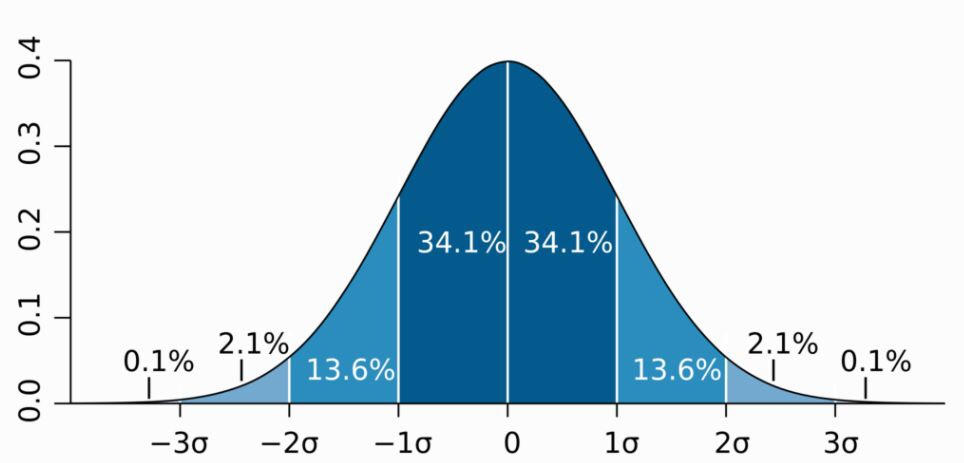正态分布为何如此常见？其真正原因是中心极限定理 (Central Limit theorem)，如果一个事物受到多种因素的影响，无论每个因素本身服从什么分布，这些因素加总后，结果的平均值就是正态分布；

正态分布值适合于各种因素叠加的情况，如果这些因素不是彼此独立的，会相互加强影响，则就不会服从正态分布。如果各种因素对结果的影响不是相加，而是相乘，则最终结果将会是对数正态分布.

最大似然估计与贝叶斯推理 (新增专题 · 待完善)

参数的意义

最大似然估计的直观解释

最大似然估计的计算

MLE

对数似然估计

最大似然估计是否总能得到精确解？

为何称作“ 『最大似然』or 『最大可能』”，而不是『最大概率』?

最小二乘参数估计和最大似然估计的结果相同的条件是什么？

贝叶斯定理

定义
举例

为何贝叶斯定理能结合先验概率？
贝叶斯推理

定义

使用贝叶斯定理处理数据分布
贝叶斯定理的模型形式
贝叶斯推断示例
何时 最大后验概率 (Maximum A Posteriori) MAP 估计 与 最大似然 (Maxmium Likelihood Estimation MLE) 估计相等？


展开全文• 中心极限定理（Central Limit Theorem）是指概率论中随机变量序列部分和分布渐近于正态分布的定理。简单而言，对于任意分布，只要随机变量之间相互独立，从这些随机变量中随机抽n个值，然后求均值，并重复足够多的...
简单解释

中心极限定理（Central Limit Theorem）是指概率论中随机变量序列部分和分布渐近于正态分布的定理。简单而言，对于任意分布，只要随机变量之间相互独立，从这些随机变量中随机抽n个值，然后求均值，并重复足够多的次数后，这些均值服从正态分布！

核心核心要点：（1）任意分布；（2）随机变量之间相互独立；（3）均值；（4）重复次数足够多

下图来自 Central limit theorem，wiki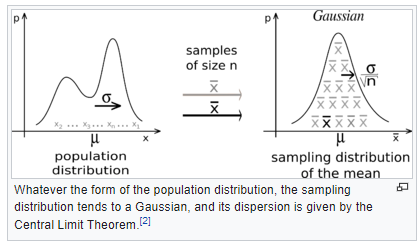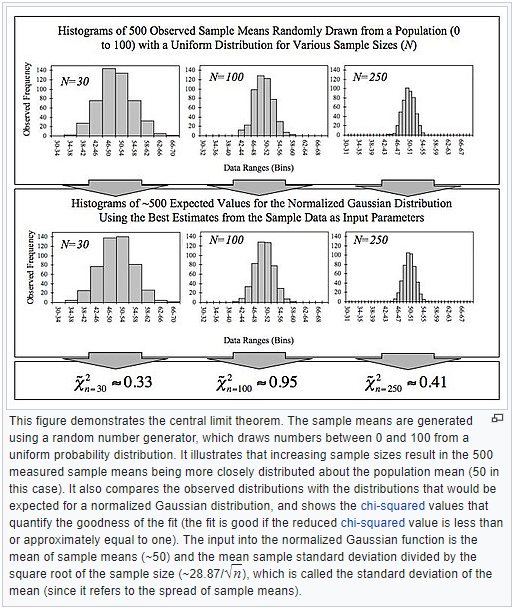扩展阅读

copy from: 中心极限定理的最最通俗解释

中心极限定理

在适当的条件下，大量相互独立随机变量的均值经适当标准化后依分布收敛于正态分布。每次从这些总体中随机抽取 n 个抽样，一共抽 m 次。 然后把这 m 组抽样分别求出平均值， 这些平均值的分布接近正态分布。

中心极限定理告诉我们，当样本量足够大时，样本均值的分布慢慢变成正态分布，就像下图：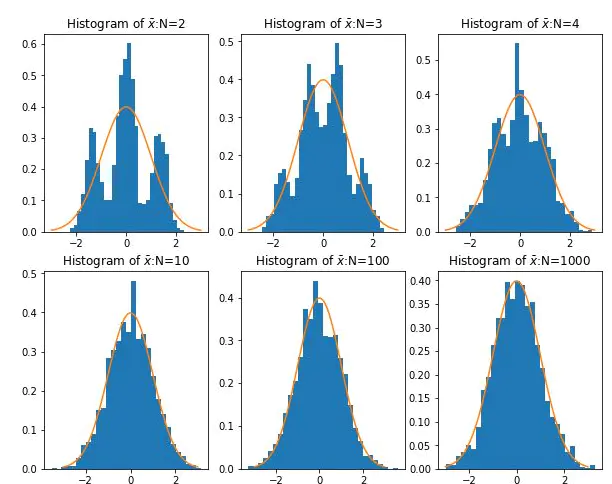实例：抽取1000组数据

我们在生产的随机数中，一次抽取50个作为一组并计算它的平均值，共抽取1000次，得到1000个平均值，然后通过seaborn的distplot看着1000个数值的分布。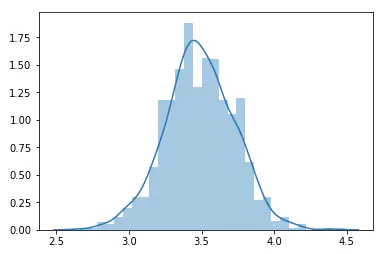重要性和注意点

当采样的数量接近无穷大时，我们的抽样分布就会近似于正态分布。这个统计学基础理论意味着我们能根据个体样本推断所有样本。结合正态分布的其他知识，我们可以轻松计算出给定平均值的值的概率。在理论上保证了我们可以用只抽样一部分的方法，达到推测研究对象统计参数的目的。

其中要注意的几点：

总体本身的分布不要求正态分布
掷一个骰子是平均分布，最后每组的平均值也会组成一个正态分布。

样本每组要足够大，但也不需要太大
取样本的时候，一般认为，每组大于等于30个，即可让中心极限定理发挥作用。

作者：statr
链接：https://www.jianshu.com/p/7e0597c0200a
来源：简书
著作权归作者所有。商业转载请联系作者获得授权，非商业转载请注明出处。

其他参考资料

（1）彻底理解中心极限定理——最重要的统计定理之一

（2）怎样理解和区分中心极限定理与大数定律？

（3）从中心极限定理的模拟到正态分布


展开全文• 你差点就赢了！首先，请注意在同一轴上绘制两个柱状图：plt.hist(avg[0:])以及^{pr2}$因此，您可以在直方图上绘制法线...在我还建议，既然您已经导入了scipy.stats，那么不妨使用该模块中的正态分布，而不是自己编写... 你差点就赢了！首先，请注意在同一轴上绘制两个柱状图：plt.hist(avg[0:])以及^{pr2}$因此，您可以在直方图上绘制法线密度，您正确地用normed=True参数对第二个图进行了标准化。但是，您也忘记了将第一个直方图标准化(plt.hist(avg[0:]), normed=True)。在我还建议，既然您已经导入了scipy.stats，那么不妨使用该模块中的正态分布，而不是自己编写pdf。在把这些放在一起，我们有：import numpy as npimport matplotlib.pyplot as pltimport scipy.stats as stats# 1000 simulations of die rolln = 10000avg = []for i in range(1,n):a = np.random.randint(1,7,10)avg.append(np.average(a))# CHANGED: normalise this histogram tooplt.hist(avg[0:], 20, normed=True)zscore = stats.zscore(avg[0:])mu, sigma = np.mean(avg), np.std(avg)s = np.random.normal(mu, sigma, 10000)# Create the bins and histogramcount, bins, ignored = plt.hist(s, 20, normed=True)# Use scipy.stats implementation of the normal pdf# Plot the distribution curvex = np.linspace(1.5, 5.5, num=100)plt.plot(x, stats.norm.pdf(x, mu, sigma))这给了我以下的情节：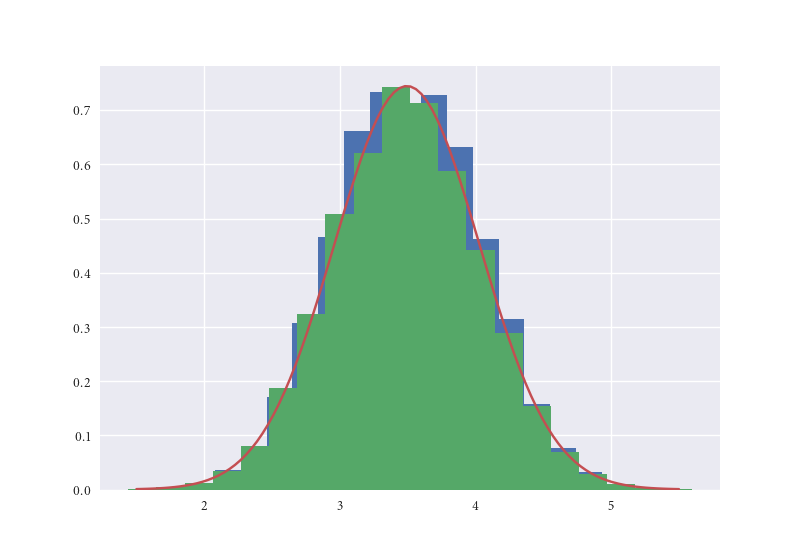编辑在你的评论中：我在np.linspace中如何选择1.5和5.5是否可以在非标准化直方图上绘制正常核？在去问q1。首先，我通过眼睛选择了1.5和5.5。在绘制柱状图之后，我看到柱状图的区域在1.5到5.5之间，所以这就是我们想要绘制正态分布的范围。在选择这一范围的一种更具纲领性的方法是：x = np.linspace(bins.min(), bins.max(), num=100)至于问题2，是的，我们可以达到你想要的。但是，你应该知道，我们根本就不会绘制概率密度函数。在在绘制直方图时删除normed=True参数后：x = np.linspace(bins.min(), bins.max(), num=100)# Find pdf of normal kernel at mumax_density = stats.norm.pdf(mu, mu, sigma)# Calculate how to scale pdfscale = count.max() / max_densityplt.plot(x, scale * stats.norm.pdf(x, mu, sigma))这给了我以下的情节：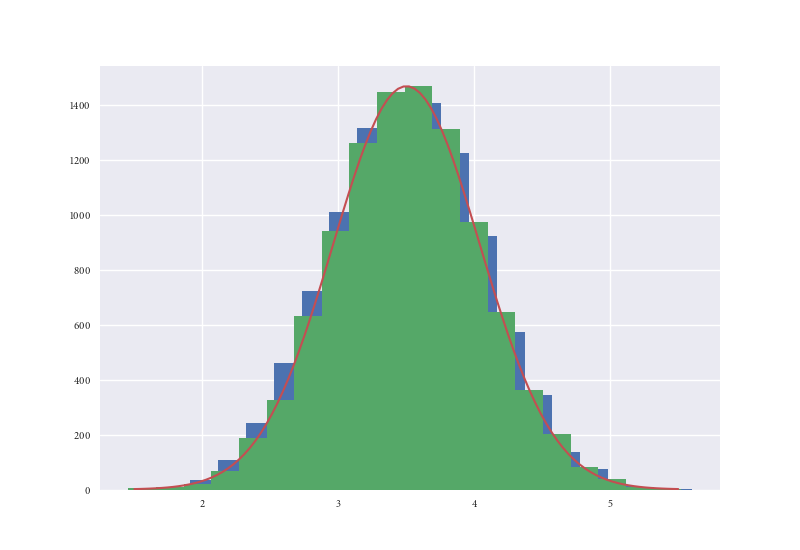展开全文•  参考文献指出了利用均匀分布生成正态分布的方法，即中心极限定理之独立同分布。 设随机变量相互独立，服从[0,1]区间上的均匀分布。则随机变量   当n足够大时，新的随机变量Y 满足标准正态分布。 但实际...


多数编程语言只提供一个生成[0,1] 区间上的均匀分布数组的函数。本人的目的在于介绍如何生成一个正态分布的随机数组

参考文献指出了利用均匀分布生成正态分布的方法，即中心极限定理之独立同分布。

设随机变量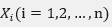相互独立，服从[0,1]区间上的均匀分布。则随机变量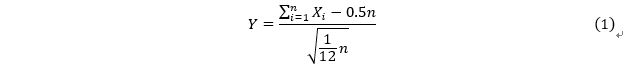当n足够大时，新的随机变量Y 满足标准正态分布。

但实际上，标准正态分布不一定能满足要求，也许需要的是满足正态分布（a，b）的随机数组。

这时就应该进行一些必要的运算。

对于均值，只需要对最终结果进行相加或者相减

对于方差的变换可以简单的将结果乘上一个系数

由正态分布的定义出发，可以证明若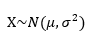则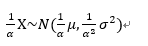已知随机变量X  服从参数为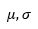的正态分布，则随机变量X 概率密度函数为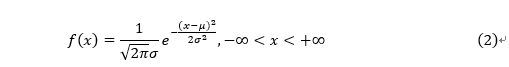令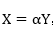，则有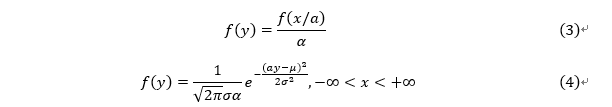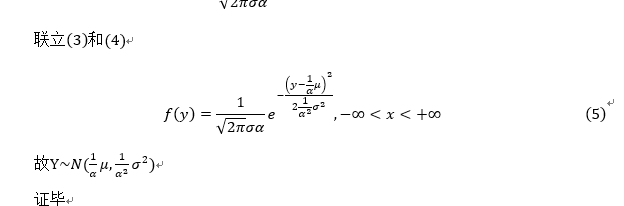接下来，我们通过C++实现这一过程

//该程序用于实现生成一组正态分布的随机数
//作者cclplus 我的邮箱是maxwell970710@gmail.com 如有问题可以发送到我的邮箱，我会尽可能为您解答
#include "stdafx.h"
#include <iostream>
#include <cmath>
#include <ctime>
using namespace std;
double rand_normal(double r_me, double sd);//返回一个方差为r_me,标准差为sd的随机数
int main(){
double ans;
double r_mean, r_sd;
int n;
cout << "请依次输入所需要正态分布随机数的均值和标准差" << endl;
cin >> r_mean >> r_sd;
cout << "请输入需要随机数的个数" << endl;
cin >> n;
for (int i = 0; i < n; i++) {
ans = rand_normal(r_mean, r_sd);
cout << "随机数：" << ans << endl;
}
return 0;
}
double rand_normal(double r_me, double sd) {
int  i;
const int normal_count = 360;//样本数目采用360个
double ccl_num, ccl_s;
double ccl_ar[normal_count];
ccl_num = 0;
for (i = 0; i < normal_count; i++) {
ccl_ar[i] =rand() % 1000/ (double)999;//生成一个[0,1]的均匀分布
ccl_num += ccl_ar[i];
}
ccl_num -= ((double)normal_count*0.5);//减去0-1均匀分布的均值
ccl_s = 1.0*(double)normal_count / 12.0;//0-1分布的方差为1/12
ccl_s = sqrt(ccl_s);
ccl_num /= ccl_s;//此时ccl_num接近标准正态分布的一个子集
ccl_num *= sd;
ccl_num += r_me;
return ccl_num;
}


【参考文献】

王桂松，张忠占，程维虎等 概率论与数理统计.[M]2014 110-110 140-141
展开全文• 于是乎我就开始琢磨用中心极限定理去简单验证一下”正态分布“。1-工具工具：当然是用的Python啦，嘿嘿。功能强大~2-前期储备知识1) 切尔雪夫不等式，设随机变量X具有数学期望,方差则对任意正数ε，不等式成立。...
• 中心极限定理： 设随机变量序列{Xi}\{X_i\}相互独立，具有相同的期望和方差，即E(Xi)=μ,D(Xi)=σ2E(X_i)=\mu, D(X_i)=\sigma^2，令Yn=X1+...+Xn, Zn=Yn−E(Yn)D(Yn)√=Yn−nμn√σY_n=X_1+...+X_n,~Z_n=\frac{Y_...
• 0-前言 ...于是乎我就开始琢磨用中心极限定理去简单验证一下”正态分布“。 1-工具 工具：当然是用的Python啦，嘿嘿。功能强大~ 2-前期储备知识 1） 切尔雪夫不等式， 设随机变量X具有数学期...
• 中心极限定理：在任意分布的总体中（定义良好，有均值，有标准差）抽取N次样本(样本容量越大)，求出每次抽取的样本的均值(或者求和等)，N次样本均值频率分布近似正态分布。 分布（密度函数） 总体分布 均值 方差...
• 正态分布 定义 正态分布（英语：normal distribution）又名高斯分布（英语：Gaussian distribution），是一个非常常见的连续概率分布。正态分布在统计学上十分重要，经常用在自然和社会科学来代表一个不明的随机...
• 1.了解什么是Machine learning 机器学习(Machine Learning, ML)是一门多领域交叉学科，涉及概率论、统计学、逼近论、凸分析、...2.学习中心极限定理，学习正态分布，学习最大似然估计 3.推导回归Loss functio...
• 中心极限定理的模拟到正态分布 2010/05/09优化与模拟、推荐文章、统计图形、统计推断R语言、SAS、Shapiro检验、中心极限定理、动画、密度曲线、数学假设条件、样本均值、样本量、正态分布、泰勒展开、直方图、...
• 正态分布，也称常态分布，又名高斯分布。正态曲线呈钟型，两头低，中间高，左右对称因其曲线呈钟形，因此人们又经常称之为钟形曲线。若随机变量X服从一个数学期望为μ、方差为σ^2的正态分布，记为N(μ，σ^2)。其...
• 中心极限定理的模拟到正态分布 谢益辉 关键词：R 语言; SAS; Shapiro 检验; 中心极限定理; 动画; 密度曲线; 数学假设条件; 样本均值; 样本量; 正态分布; 泰勒展开; 直方图; 统计模拟; 钟形曲线; ...
• 之前看概率论的书，谈到正态分布的第一个反应就是那个看起来很随便的突起的曲线，以及现实生活中好像有很多东西都符合正态分布，除此之外就再没有别的理解了，直到最近看书才慢慢体会到了概率中藏着的很多秘密。...
• 中心极限定理说明很多独立随机变量的和近似服从正态分布。 二，在具有相同方差的所有可能的概率分布中，正态分布在实数上具有最大的不确定性。 因此，我们可以认为正态分布是对模型加入的先验知识量最少的分布。 ...
• 正态分布： 一种概率密度函数 ...中心极限定理： 随着样本容量趋于无穷或者非常大的时候，得到的分布近似正态分布（不论是样本均值，或者是样本的和或者是其他）（有些甚至连概率都不知道） ...
• 数据分析中正态分布的作用 正态分布的作用 正态分布准确地说什么是标准离差?它用最标准方式表达的期望值(标准差)的离差。 第一步是收集一系列教据和决定你的期望值是多少。 例如找们讨论的抛两个般子的情况.你...数据分析
• 在本示例中，选择a=0，b=1，这个均匀分布的方差等于（1/12）。 #定义高斯采样函数 def gauss_rand(): n = 100; #均匀产生100个0~1之间的随机数 u = np.random.uniform(0,1,n) r = (sum(u) - n * 0.5) / np...
• 殊不知，这问题的本身就是错误的，并不是样本大，就一定要服从正态分布。我们可以轻易举出一个反例来说明这个问题。比方说就用1-1000这一千个（甚至更多）自然数，组成一个样本，那么这个样本的分布就不是正态分布，...
• 概率论中有很多的近似计算让学生感到很困惑，不知道什么时候该用，省么时候不该用
• 1.概率均匀分布（C语言）#include &lt;stdio.h&gt; #include &lt;stdlib.h&gt; #include &lt;time.h&gt; int main( int argc, char **argv) { int i=0, v, mod, n; /* srand函数是...
• import numpy as np import matplotlib.pyplot as plt ...#Show Normal Distribution/正态分布展示 def demo1(sample_number): mu ,sigma = 0, 1 np.random.seed(0) s = np.random.normal(mu, sigma, sample_numb...
• 当 YYY 较大时用正态分布函数拟合求 [A−nμnσ,B−nμnσ]\left[\frac {A-n\mu}{ \sqrt n\sigma},\frac {B-n\mu}{\sqrt n\sigma}\right][n​σA−nμ​,n​σB−nμ​] 的积分，用自适应辛普森，或者C+...